Bihar Board 12th Maths Important Questions Long Answer Type Part 6

BSEB Bihar Board 12th Maths Important Questions Long Answer Type Part 6 are the best resource for students which helps in revision.

Bihar Board 12th Maths Important Questions Long Answer Type Part 6

Vector Algebra

Question 1.
For any three vectors a, b and c
$$(\vec{a}+\vec{b})+\vec{c}=\vec{a}+(\vec{b}+\vec{c})$$
Solution:
Let the vectors $$(\vec{a}$$, $$(\vec{b}$$ and $$(\vec{c}$$ be represented by $$\overrightarrow{P Q}, \overrightarrow{Q R}$$ and $$\overrightarrow{RS}$$ respectively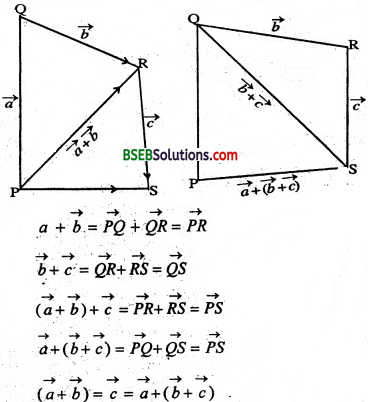The associative property of vector addition enables us to write the sum of three vectors $$\vec{a}, \vec{b}, \vec{c}$$ as $$\vec{a}+\vec{b}+\vec{c}$$ without using brackets.Three Dimensional Geometry

Question 1.
Find the angle .between the pair of tines given by s
and $$\vec{r}=\dot{3} \hat{i}+2 \hat{j}-4 \hat{k}+\lambda(\hat{i}+2 \hat{j}-2 \hat{k})$$ and $$\vec{r}=5 \hat{i}-2 \hat{j}+\mu(3 \hat{i}+2 \hat{j}-6 \hat{k})$$
Solution:
Here $$\overrightarrow{b_{1}}=\hat{i}-2 \hat{j}+2 \hat{k}$$ and $$\overrightarrow{b_{2}}=3 \hat{i}+2 \hat{j}+6 \hat{k}$$
The angle θ between the two lines is given by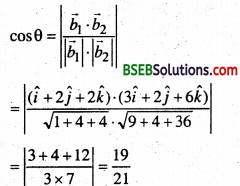Hence θ = cos-1($$\frac{19}{21}$$)

Question 2.
Find the vector equation of the plane passing through the intersection of the planes $$\vec{r} \cdot(\hat{i}+\hat{j}+\hat{k})=6 \text { d } \vec{r} \cdot(2 \hat{i}+3 \hat{j}+4 \hat{k})=-5$$ and the point (1,1,1).
Solution:
Here $$\overrightarrow{n_{1}}=\hat{i}+\hat{j}+\hat{k}$$ and $$\overrightarrow{n_{2}}=2 \hat{i}+3 \hat{j}+4 \hat{k}$$
and d1 = 6 and d2 = -5
Hence using the relation $$\vec{r} \cdot\left(\vec{n}_{1}+\lambda \vec{n}_{2}\right)=d_{1}+\lambda d_{2}$$
We get $$\vec{r} \cdot[\hat{i}+\hat{j}+\hat{k}+\lambda(2 \hat{i}+3 \hat{j}+4 \hat{k})]=6-5 \lambda$$
or, $$\vec{r} \cdot[(1+2 \lambda) \hat{i}+(1+3 \lambda) \hat{j}+(1+4 \lambda) \hat{k}]=6-5 \lambda$$………………(i)
where, λ is some real number.
Takipg $$\vec{r} \cdot x \hat{i}+y \hat{j}+2 \hat{\hat{k}}$$,we get
(x$$\hat{i}$$ + y$$\hat{j}$$ + z$$\hat{k}$$)-[(1+2^)$$\hat{i}$$ +(1 + 3A.)$$\hat{j}$$ + (l + 4A.)$$\hat{k}$$] = 6 – 5λ
or, (1 + 2λ)x + (1 + 3λ)y + (1 + 4λ)z = 6 – 5λ
or, (x + y + z – 6) + λ(2x + 3y + 4z + 5) = 0 ………………………(ii)
Given that the plane passes through the point (1,1, 1) it must satisfy (2).
i.e. (1+ 1+ 1-6) + λ(2+3+4+5) = 0
or λ = $$\frac{3}{14}$$
Putting the value of λ in (1), we getwhich is the required vector equation of the plane.Question 3.
Find the shortest distance between the l1 and l2 whose vector equation are
$$\vec{r}=\hat{i}+\hat{j}+\lambda(2 \hat{i}+\hat{j}+\hat{k})$$
and $$\overrightarrow{\boldsymbol{r}}=\mathbf{2} \hat{i}+\hat{j}-\hat{k}+\mu(3 \hat{i}-5 \hat{j}+2 \hat{k})$$
Solution:
Comparing (i) and (ii) with $$\vec{r}=\overrightarrow{a_{1}}+\lambda \overrightarrow{b_{1}}$$ and $$\vec{r}=\overrightarrow{a_{2}}+\mu \overrightarrow{b_{2}}$$ respectively.

We get $$\overrightarrow{a_{1}}$$ = $$\hat{i}$$ + $$\hat{j}$$ $$\overrightarrow{b_{1}}$$ = 2$$\hat{i}$$ – $$\hat{j}$$ + $$\hat{k}$$
$$\overrightarrow{a_{2}}$$ =2$$\hat{i}$$ + $$\hat{j}$$ – $$\hat{k}$$ and & $$\overrightarrow{b_{2}}$$ = 3$$\hat{i}$$ – 5$$\hat{j}$$ + 2$$\hat{k}$$
and $$\overrightarrow{b_{2}} \times \overrightarrow{b_{2}}=(2 \hat{i}-\hat{j}+\hat{k}) \times(3 \hat{i}-5 \hat{j}+2 \hat{k})$$
Therefore, $$\overrightarrow{a_{2}}-\overrightarrow{a_{1}}=\hat{i}-\hat{k}$$
$$\left|\begin{array}{lll} \hat{i} & \hat{j} & \hat{k} \\ 2 & -1 & 1 \\ 3 & -5 & 2 \end{array}\right|$$ = 3$$\hat{i}$$ – $$\hat{j}$$ – 7$$\hat{k}$$
$$\left|\overrightarrow{b_{1}} \times \vec{b}_{2}\right|=\sqrt{9+1+49}=\sqrt{59}$$
Hence the shortest distance between the given lines is given by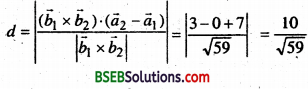Question 4.
Find the vector and the cartesian equations of the Hoe through the point (5, 2, -4) and which Is parallel to the vectors $$3 \hat{i}+2 \hat{j}-8 \hat{k}$$
Solution:
We have
$$\overrightarrow{a}$$ = 5$$\hat{i}$$ + 2$$\hat{j}$$ – 4$$\hat{k}$$ and $$\overrightarrow{b}$$ = 3$$\hat{i}$$ +2$$\hat{j}$$ – 8$$\hat{k}$$
Therefore, the vector of the line is ‘
$$\overrightarrow{r}$$ = 5$$\hat{i}$$+2$$\hat{j}$$-4$$\hat{k}$$ + λ(3$$\hat{i}$$+2$$\hat{j}$$-8$$\hat{k}$$)
Now, $$\overrightarrow{r}$$ is the position vector of any point p (x,y, z) on the line.

Therefore x$$\hat{i}$$ + y$$\hat{j}$$ + 2$$\hat{k}$$ = 5$$\hat{i}$$ + 2$$\hat{j}$$ -4$$\hat{k}$$ + 2(3$$\hat{i}$$ + 2 $$\hat{j}$$– 8$$\hat{k}$$)
= (5 + 3λ)$$\hat{i}$$ + (2 + 2λ)$$\hat{j}$$ + (-4 – 8λ)$$\hat{k}$$
Eliminating λ, we get
$$\frac{x-5}{3}=\frac{y-2}{2}=\frac{z+4}{-8}$$
which is the equation of the line is cartesian form.

Question 5.
Find the angle between the two planes 2x + y – 2z = 5 and 3x – 6y – 2z = 7 using vector method.
Solution:
The angle between two planes is the angle between their normals. From the equation of the planes the normal vectors are
$$\vec{N}_{1}$$ = 2$$\hat{i}$$ + $$\hat{j}$$ – 2$$\hat{k}$$ and $$\vec{N}_{2}$$ = 3$$\hat{i}$$ – 6$$\hat{j}$$ – 2$$\hat{k}$$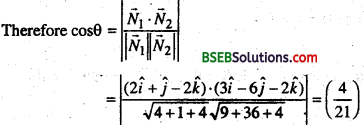Hence θ = cos-1( $$\frac{4}{21}$$ )Question 6.
Find the angle between the line
$$\frac{x^{\circ}+1}{2}=\frac{y}{3}=\frac{z-3}{6}$$ and the plane 10x + 2y – 112 = 3
Solution:
Let θ be the angle between the line and the normal to the plane .
Converting the given equations into vector form we have
$$\vec{r}$$ = (-$$\hat{i}$$ + 3$$\hat{k}$$ + (2$$\hat{i}$$ + 3$$\hat{j}$$ – 6$$\hat{k}$$ )
and $$\vec{r}$$ = (10$$\hat{i}$$ + 2$$\hat{j}$$ + (2$$\hat{i}$$ – 11$$\hat{k}$$ ) = 3
Here $$\vec{b}$$ = (2$$\hat{i}$$ + 3$$\hat{j}$$ – 6$$\hat{k}$$ and $$\vec{n} 10 \hat{i}+2 \hat{j}-11 \hat{k}$$im

Question 7.
Find the equation of the plane that contains the point (1, -1,2) and is perpendicular to each of tfie planes 2x + 3y – 2z – 5 and x + 2y-3z = 8.
Solution:
The equation of the plane containing the given point is
A(x-1) + B(y+1) + C(z-2) = 0 ….(i)
Applying the condition of perpendicularly to the plane given in eqn. (i) with the planes
2x+3y -2z = 5 and x + 2y – 3z = 6 we have
2A + 3B-2C= 10 and A + 2B – 3C = 0
Solving these equations. We find A =-5C and B = 4C.
Hence the required equation is
– 5C (x – 1) + 4C (y+ 1) + C(z – 2) = 0
i.e. 5x – 4y – z = 7

Question 8.
Find the coordinates of the point where the line through the point A (3,4,1) and B (5,1,6) crosses the xy-plane.
Solution:
The vector equation of the line through the points A and B is
$$\vec{r}=3 \hat{i}+4 \hat{j}+\hat{k}+\lambda[(5-3) \hat{i}+(1-4) \hat{j}+(6-1) \hat{k}]$$
i.e. $$\overrightarrow{\boldsymbol{r}}=3 \hat{i}+4 \hat{j}+\hat{k}+\lambda(2 \hat{i}-3 \hat{j}+5 \hat{k})$$ ….(i)
Let P be the point where the line AB crosses the xy-plane. Then the position vector of the point P is of the form x$$\hat{i}$$ + y $$\hat{i}$$
This point must satisfy the eqn. (i)
i.e. x$$\hat{i}$$ + y$$\hat{j}$$ = (3 + 2λ)$$\hat{i}$$ + (4 – 3λ)$$\hat{j}$$ + (1 + 5λ)$$\hat{k}$$
Equating the like coefficient of $$\hat{i}$$ + $$\hat{j}$$ and $$\hat{k}$$.
We have
x = 3 + 2λ
y = 4 – 3λ
0 = 1 + 5λ
Solving the above equations, we get
x = $$\frac{13}{5}$$ and y = $$\frac{23}{5}$$
(13 23 ‘t
Hence the coordinates of the required point are ($$\frac{13}{5}$$, $$\frac{23}{5}$$, 0)

Question 9.
Find the distance of the plane 2x – 3y + 4z – 6 = 0 from the origin.
Solution:
Since the direction ratio of the normal to the plane are 2, -3,4 the direction cosines of it are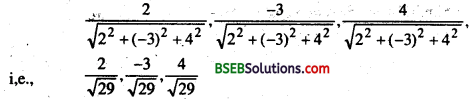im
Hence dividing the equation 2x – 3y + 4z – 6 = 0.
2x – 3y + 4s = 6 throughout by $$\sqrt{29}$$ we get
$$\frac{2}{\sqrt{29}} x+\frac{-3}{\sqrt{29}} y+\frac{4}{\sqrt{29}} z=\frac{6}{\sqrt{29}}$$
This is of the form fct +my + nz-d. Where d is the distance of the plane from the origin. So the distance of the plane from the orign is $$\frac{6}{\sqrt{29}}$$Question 10.
A line makes angle α, β, γ and δ with the digonals of a cube, pro e that
cos2 α + cos2 β + cos2 γ + cos2 δ = $$\frac{4}{3}$$
Solution:
A cube is a rectangular parallelopiped having equal length, breadth and height.Let OADBFEGC be the cube with each side of length a units.
The four diagonals are OE, AF, BG and CD.
The direction cosines of the diagonal OE which is the line joining two point O and E are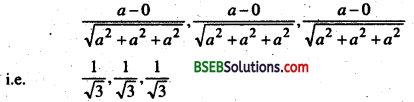Similarly, the direction cosines of AF, BG and CD are.Let, m, n be the direction cosines of the given line which makes angles α, β, γ, δ with OE, AF, BG, CD respectively.
Then cos α = $$\frac{1}{\sqrt{3}}$$(l + m + n)
cos β = $$\frac{1}{\sqrt{3}}$$(-l + m + n)
cos γ = $$\frac{1}{\sqrt{3}}$$(l – m + n)
c0s δ = $$\frac{1}{\sqrt{3}}$$(l + m-n)
cos2 α + cos2 β + cos2 γ + cos2 δ
= $$\frac{1}{3}$$ [(l + m +n)2 +(-l + m + n)2 + (l – m + n)2 +(l + m – n)2]
= $$\frac{1}{3}$$[4(l2 + m2 + n2)] = $$\frac{4}{3}$$(asl2 + m2 + n2 = 1)

Linear Programming

Question 1.
Minimize
Z = -3x + 4y Subject to constrants ’ x +2y ≤ 8,3x + 2y ≤ 12 ,x ≥ 0,y ≥ 0.
Solution:
x + 2y = 8 ⇒ $$\frac{x}{8}+\frac{y}{4}$$ = 1
3x + 2y = 12 ⇒ $$\frac{x}{4}+\frac{y}{6}$$ = 1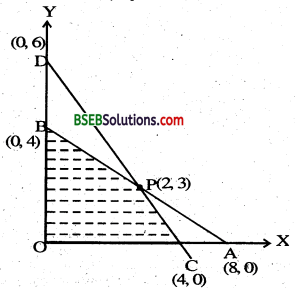∴ the feasible region is
OCPBO
The coordinates of. P can be determined by solving x + 2y = 8, 3x +2y =12.
∴ coordinates of P = (2, 3)
Z at O (0,0) = – 3x + 4y = 0
Z at C (4,0) = – 3 x 4 = -12
Z at P (2, 3) = – 3 x 2 + 4 x 3 = 6
ZatB (0,4) = 4 x 4= 16
Hence the minimum value of Zis -12 at the point C (4,0).Question 2.
Solve the following linear programming problem graphically :
Maximize Z = 4x + y…………. (i)
( Subject to the constraints
x + y ≤ 50 ….(ii)
3x + y ≤ 90 ……….(iii)
x ≥ 0, y ≥ 0 …….(iv)
Solution:
The shaded region in fig. is the feasible region determined by the system of constraints (ii) to (iv). We observe that the feasible region OABC is bounded. So we now use comer point method to determine the maximum value of Z. The coordinates of the comer points O, A, B and C are (0,0), (30,0), (20, 30) and respectively.
Now we evaluate Z at each corner point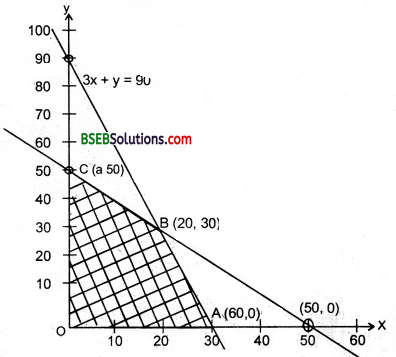table
Hence, maximum value of Z is 120 at the point (30, 0)

Question 3.
A Dietician has to develop a special diet using two foods p and q Each packet (containing 30 g) of food P contains 12 unit of calcium, 4 units of iron, 6 units of cholesterol and 6 units of vitamin A. Each packet of the same quantity of food Q contains 3 units of calcium, 20 units of iron, 4 units c f chol e s te r [‘& 3 units of’ – min A, The d let requires at least 240 units of calcium, atleast 460 units of iron and atriiost 300 units of cholesterol. How many packets of each food should be used to minimise the amount of vitamin A In the diet 2 What is the minimum amount of viiamiiin A ?
Solution:
Let x and y be the number of packets of food P and Q respectively. Obviously x ≥ 0, y ≥ 0. Mathematical formulation of the given problem is as follows:

Minimise
Z = 6.r+ 3v (vitamin A)
Subject to the constraints
12x+ 3y ≥ 240 (constraint on calcium),
4x + y ≥ 80
4x + 20v ≥ 460 (constraint on iron), x + 5v >115
6x + 4y ≥ 3 w (constraint on cholesterol),
3* + 2y ≤ 150 x ≥ 0, y ≥ 0
Let us graph the inequalities (i) to (iv).
The feasible region (shaded) determined by the constraints (i) to (iv) is show in figure, and note that is bounded.The coordinates of the comer points L, M and N are (2,7,2), (15,20) and (40, 15) respectively. Let us evaluate Z at these points.
table
From the table, we find that Z is minimum at the point (15,20). Hence, the amount of vitamin A under the constraints given in the problem will be minimum, if 15 packets of food P and 20 Packets of food Q are used in the special diet. The minimum amount of vitamin A will be 150 units.Probability

Question 1.
A die is thrown three times, Events A and B are fined as below:
A : 4 on the third throw
B : 6 on the first and 5 on the Second throw
Find the probability of A given that B has already occurred,
Solution:
The sample space has 216 outcomes.B ={(6, 5, 1), (6. 5, 2). (6.5. 3), (6,5,4), (6,5,5), (6,5, 6)} and A ∩ B = {(6,5,4)}Question 2.
An unbased die is thrown twice. Let the event A be odd number on the first throw and B the event odd number on the second throw. Check the independence of the events A and B.
Solution:
If all the 36 elementary events of the experiment are considered to be equally likely, we have
P(A) = $$\frac{18}{36}=\frac{1}{2}$$
P(B) = $$\frac{18}{36}=\frac{1}{2}$$
Also P (A ∩ B) = P (odd number on both throws)
= $$\frac{9}{36}=\frac{1}{4}$$
Now P(A)P(B) = $$\frac{1}{2} \times \frac{1}{2}=\frac{1}{4}$$
Clearly P (A n B) = P (A) x P (B)
Thus A and B are independent events.

Question 3.
An urn contains 10 black and 5 white balls. Two balls are drawn from the urn one after the other without replacement. ‘What is the probability that both drawn balls are black.
Solution:
Let E and F denote respectively the events that first and second ball drawn are black.
We have to find P (E ∩ F) or P (EF).
Now P (E) = P (black ball in first draw) = $$\frac{10}{15}$$
Also given that first ball drawn is black, i.e. event E has occured. Now therefore 9 black balls and five white balls left in the urn. Therefore the probability that the second ball drawn is black, given that the ball in first draw is black, is nothing the conditional probability of F given that E has occured.
i.e. p(F\E) = $$\frac{9}{14}$$
By multiplication rule of probability, we have P(E∩F) = P(E)P(F|E)
= $$\frac{10}{15} \times \frac{9}{14}=\frac{3}{7}$$

Question 4.
Ten cards numbered 1 to: 10 are placed in a box, raised up thoroughly and then one card is drawn randomly. If It is know n that the number OK the drawn card is more than 3. What is the probability that It is an even number ?
Solution:
Let A be the event the number on the card drawn is even and B be the event the number on the card drawn is greater than 3.
We have to find P (A | B).
Now, the sample space of the experiment is δ ={ 1,2, 3,4, 5,6, 7, 8,9, 10}.
A = {2,4, 6, 8,10}, B = {4,5,6, 7, 8,9,10}
Also A∩B = {4,6, 8, 10}
Also P(A) = $$\frac{5}{10}$$, P(B) = $$\frac{7}{10}$$
and P(A∩B) = $$\frac{4}{10}$$
Then P(A/B) = $$\frac{P(A \cap B)}{P(B)}$$
= $$\frac{\frac{4}{10}}{\frac{7}{10}}=\frac{4}{10} \times \frac{10}{7}=\frac{4}{7}$$Question 5.
Prove that if E and F are independent events, then so are the events E and F.
Solution:
Since E and F are independent, we have
P(E∩F) = P.E. P (F)
From the even diagram in fig. It is clear that E∩F and E∩F’ are mutually exclusive events and also .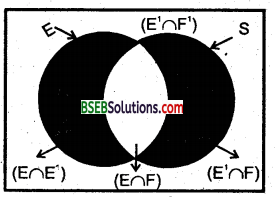E = (E ∩ F) ∪ (E ∩ F)
Therefore P (E) = P(E∩F) +(E∩F)
or, P (E∩F) = P(E) – P (E∩F)
= P (E) – P (E) P (F) (by (1))
= P(E) (1 – P(F))
= P (E) . P (F)
Hence, E and F are indepent.

Question 6.
Three coins are tossed simultaneously. Consider the event E three heads or three, tails*. F^’at least two heads’ and G ’at most two heads’ of the pairs (E, F), (E,G) and (F, G) which are independent ? Which
are dependent ?
Solution:
The same space of the experiment is given by
S= {HHH, HHT, HTH, THH, HTT, THT, TTH, TTT)
Clearly E = {HHH, TTT)
F = {HHH, HHT, HTH, THH)
and G = {HHT, HTH, THH, HTT, THT, TTH, TTT)
Also E ∩ F = {HHH},
E∩G = {TTT},
F∩G = [HHT, HTH, THH},
Therefore P(E) = $$\frac{2}{8}=\frac{1}{4}$$
P(F) = $$\frac{4}{8}=\frac{1}{2}$$
P(G) = $$\frac{7}{8}$$
and P(E∩F) = $$\frac{1}{8}$$
P(E∩G) = $$\frac{1}{8}$$
P(E∩C) = $$\frac{3}{8}$$
Also P(E)P(F) = $$\frac{1}{4} \times \frac{1}{2}=\frac{1}{8}$$
P(E)P(G) = $$\frac{1}{4} \times \frac{7}{8}=\frac{7}{32}$$
and P(F)P(G) = $$\frac{1}{2} \times \frac{7}{8}=\frac{7}{16}$$
Thus P(E∩F) = P (E)P(F)
P (E∩G) ≠ P(E).P(G)
and P (F ∩G) ≠ P(F) .P (G)
Hence, the events (F and F) are independent, and the events (E and G) and (F and G) are dependent.

Question 7.
A person has under taken a construction job. The probabilities are 0 65 that there will be strike 0 80 that construction job will be completed on time if there is no strike, and 0 32 that construction job w ill be completed on time if there is a strike. Determine the probability that the construction job will be completed on time.
Solution:
Let A be the event that the construction job will be completed on time and B be the event that there will be 9 strike. We have to find P (A).
We have
P (B) = 0.65,
P (no strike) = P(B)= 1 – P(B)
= 1 – 0.65 = 0.35
P (A | B) = 0.32
P(A IB1) = 0.80
Since events B and B’ form a partition of the sample spaces, There fore, by theorem on total probability, we have
P(A) = P(B)P(A|B)+P(B,)P(A|B’)
= 0.65 x 0.32 + 0.35 x 0.8
= 0.208 + 0.28
= 0.488
Thus, the probability that the construction job will be completed in time is 0.488.
We shall now state and prove the Baye s theorem.Question 8.
Tew eggs are drawn successively with replacement from a lot conjoining 10% defflective eggs. Find the probability that there is at least one deflective eggs.
Solution:
Let X denote the number of defective eggs in the 10 eggs drawn. Since the drawing is done with replacement the trials Bemoullitrials clearly,
X has the binomial distribution with n = 10 and P = $$\frac{10}{100}=\frac{1}{10}$$
Therefore q = 1 – p = $$\frac{9}{10}$$
Now e (at least one defective egg) = P (X ≥ 1) = 1 – P(X = 0)
= 1 – 10c0 ( $$\frac{9}{10}$$ )10
= 1 – $$\frac{9^{10}}{10^{10}}$$